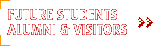1998 Technical Reports

## Robot Map Verification of a Graph World

### X. Deng, E. Milios and A. Mirzaian

Technical Report CS-1998-02

York University

April 12, 1998

Abstract

In the map verification problem, a robot is given a (possibly incorrect) map M of the world G with its position and orientation indicated on the map. The task is to find out whether this map, for the given robot position and orientation in the map, is correct for the world G. We consider the world model with a graph G=(V_G,E_G) in which, for each vertex, edges incident to the vertex are ordered cyclically around that vertex. (This holds similarly for the map M=(V_M,E_M).) The robot can traverse edges and enumerate edges incident on the current vertex, but it cannot distinguish vertices and edges from each other. To solve the verification problem, the robot uses a portable edge marker, that it can put down and pick up as needed. The robot can recognize the edge marker when it encounters it in G. By reducing the verification problem to an exploration problem, verification can be completed in O(|V_G|\times |E_G|) edge traversals (the {\em mechanical cost}) with the help of a single vertex marker which can be dropped and picked up at vertices of the graph world [DJMW1,DSMW2]. In this paper, we show a strategy that verifies a map in O(|V_M|) edge traversals only, using a single edge marker, when M is a {\em plane} embedded graph, even though G may not be (e.g., G may contain overpasses, tunnels, etc.). In general, when the map has embedded genus g (intuitively, a plane embedded graph has genus 0, and each overpass added to it may increase the embedded genus by 1), we show it can be verified with O(|E_M|) mechanical cost, using g+1 distinguishable markers.